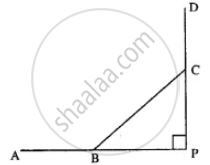# Two Alternate Sides of a Regular Polygon, When Produced, Meet at the Right Angle. Calculate the Number of Sides in the Polygon. - Mathematics

Sum

Two alternate sides of a regular polygon, when produced, meet at the right angle. Calculate the number of sides in the polygon.

#### SolutionLet number of sides of regular polygon = n

AB & DC when produced meet at P such that

∠P = 90°

∵ Interior angles are equal.

∴ ∠ABC = ∠BCD

∴ 180° - ∠ABC = 180° - ∠BCD

∴ ∠PBC = ∠BCP

But ∠P = 90° (given)

∴ ∠PBC + ∠BCP = 180° - 90° = 90°

∴ ∠PBC =∠BCP

= 1/2 XX 90° = 45°

∴ Each exterior angle = 45°

therefore 45^circ = 360^circ/"n"

n = 360^circ/45^circ

n = 8

Concept: Regular Polynomial
Is there an error in this question or solution?

#### APPEARS IN

Selina Concise Mathematics Class 8 ICSE
Chapter 16 Understanding Shapes
Exercise 16 (B) | Q 13 | Page 184